Download Presentation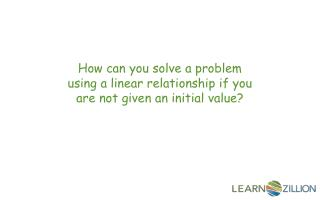How can you solve a problem using a linear relationship if you are not given an initial value?

# How can you solve a problem using a linear relationship if you are not given an initial value? - PowerPoint PPT Presentation

How can you solve a problem using a linear relationship if you are not given an initial value?. In this lesson you will learn how to solve a problem involving a linear relationship by making a table and graph.I am the owner, or an agent authorized to act on behalf of the owner, of the copyrighted work described.
Download Presentation## How can you solve a problem using a linear relationship if you are not given an initial value?

An Image/Link below is provided (as is) to download presentation

Download Policy: Content on the Website is provided to you AS IS for your information and personal use and may not be sold / licensed / shared on other websites without getting consent from its author.While downloading, if for some reason you are not able to download a presentation, the publisher may have deleted the file from their server.

- - - - - - - - - - - - - - - - - - - - - - - - - - E N D - - - - - - - - - - - - - - - - - - - - - - - - - -
Presentation Transcript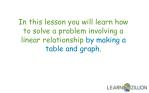In this lesson you will learn how to solve a problem involving a linear relationshipby making a table and graph.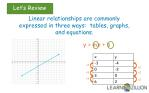Linear relationships are commonly expressed in three ways: tables, graphs, and equations.

y = mx + b

+3

+2

+2

+3

+3

+2You are going to a carnival with your friend. You know that there is a fee to get into the carnival, and then you also pay to go on rides.

Your friend paid \$5.50 and rode 2 rides.

Each ride costs \$0.75.

How much will it cost to pay the entry fee and ride 8 rides?

Constant rate of change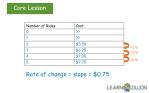+0.75

+0.75

Rate of change = slope = \$0.75

+0.75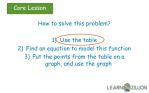How to solve this problem?

• Use the table
• Find an equation to model this function
• Put the points from the table on a graph, and use the graph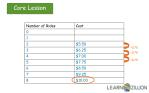+0.75

+0.75

+0.75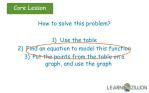How to solve this problem?

• Use the table
• Find an equation to model this function
• Put the points from the table on a graph, and use the graphWork backwards

\$4.00

-0.75

\$4.75

-0.75

c = \$0.75r + \$4.00

c = \$0.75(8) + \$4.00 = \$10.00How to solve this problem?

• Use the table
• Find an equation to model this function
• Put the points from the table on a graph, and use the graph+0.75

+0.75

Rate of change = slope = \$0.75 = ¾

+0.75(8, \$10.00)

(5, \$7.75)

Cost (in dollars)

(4, \$7.00)

(3, \$6.25)

(2, \$5.50)

Number of ridesIn this lesson you have learned how to solve a problem involving a linear relationshipby making a table and graphing.A 2-mile cab ride costs \$5.25. A 5-mile cab ride costs \$10.50. How much does a 4.2-mile cab ride cost, if the cost of the cab is calculated by the mile?A water jug with 3 cups of water in it weighs 2.25 pounds. With 5 cups of water it weighs 3.25 pounds. How much does the water jug weigh when empty?Sean likes to eat cookies. He eats 3 cookies per day. Five days after opening a box of cookies, there are 24 cookies left in the box. How many cookies were in the box at the time it was opened?